# Units - math word problems

#### Number of problems found: 4431

• Percentage increaseWhat is the annual percentage increase in the city when the population has tripled in 20 years?
• Fruit importsCarriers import 18,324 kg of oranges from Spain. It was 16,857 kg less than mandarins and 2,645 kg more than bananas. a) how many kg did they bring mandarins? b) how many kg did bananas bring? c) how much total fruit?
• Age of familyThe age of father and son is in the ratio 10: 3. The age of the father and daughter is in a ratio of 5: 2. How old are a father and a son if the daughter is 20?
• Magnified cubeIf the lengths of the cube's edges are extended by 5 cm, its volume will increase by 485 cm3. Determine the surface of both the original and the magnified cube.
• AL wireWhat is the weight of an aluminum wire 250 m long with a diameter of 2 mm, if the density of aluminum is p = 2700 kg/m cubic. Determine to the nearest gram.
• Trapezoidal prism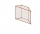Calculate the volume of a prism with a trapezoidal base with side a = 6dm, side c = 4 dm, height of the prism = 8dm. The height of the trapezoid is va = 3dm.
• Brass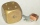Brass is an alloy of copper and zinc in a ratio of 3: 2. How many grams does a component that required 270 g of copper weigh?
• Runcated pyramid teapot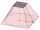The 35 cm high teapot has the shape of a truncated pyramid with the length of the edge of the lower square base a=50 cm and with the edges of the rectangular base b: 20 cm and c: 30 cm. How many liters of water will fit in the teapot?
• Central angleWhat is the length of the arc of a circle with a diameter of 46 cm, which belongs to a central angle of 30°?
• DriversDriver Franta and driver Karel are friends. They leave the start-stop both at the same time in the afternoon - at 13.30. When will they meet again if Franta goes around his route in 50 minutes and Karel in 40 minutes?
• Cyclist 12What is the average speed of a cycle traveling at 20 km in 60 minutes in km/h?
• Ditch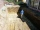The excavator dug 40% of the excavation in 2 hours 20 minutes. How long does it take him to dig the whole ditch?
• Hectoliters of waterThere are 942 hectoliters of water in a cylindrical tank with an inner diameter of 6 m. The water reaches two thirds of the depth of the tank. Calculate its depth.
• Viewing angleThe observer sees a straight fence 60 m long at a viewing angle of 30°. It is 102 m away from one end of the enclosure. How far is the observer from the other end of the enclosure?
• The convoy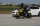During a military exercise, the convoy left the camp at 5 hours at a speed of 40 km/h. Half an hour later, a motorcycle clutch was sent at a speed of 70 km/h. How long before the motorcycle clutch reaches the column?
• Interior angles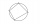In a quadrilateral ABCD, whose vertices lie on some circle, the angle at vertex A is 58 degrees and the angle at vertex B is 134 degrees. Calculate the sizes of the remaining interior angles.Adam has half the money in his right pocket than in his left pocket. If he transferred 40 crowns from the left pocket to the right, he would have the same in both pockets. Calculate how many crowns does Adam has in his left pocket more than in his right?From the observation tower at a height of 105 m above sea level, the ship is aimed at a depth angle of 1° 49´. How far is the ship from the base of the tower?The regular pentagonal prism is 10 cm high. The radius of the circle of the described base is 8 cm. Calculate the volume and surface area of the prism.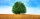The shadow of a 1 m high pole thrown on a horizontal plane is 0.8 m long. At the same time, the shadow of a tree thrown on a horizontal plane is 6.4 m. Determine the height of the tree.